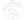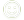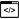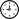Courses Details
Analyzing Data with ExcelCourse Code 20779Duration 3 Days
Request Group Training
Course Overview
The main purpose of the course is to give students the ability to add BI techniques to Excel data analysis. The course goes beyond the capabilities of tables and charts and uses Pivot Charts, the Excel Data Model, and Power BI.
Course Schedule
Target Audience
##### The course will likely be attended by SQL Server report creators who are interested in alternative methods of presenting data
Course Prerequisites

Before attending this course, students must have:

• Basic knowledge of the Microsoft Windows operating system and its core functionality.
• Working knowledge of relational databases.
• Extensive knowledge of Excel spreadsheets including formulas, charts, filtering, sorting, and sub-totals.
• Basic knowledge of data analytics equivalent to having attended course 10994.
Expected Accomplishments

After completing this course, students will be able to:

• Explore and extend a classic Excel dashboard.
• Explore and extend an Excel data model.
• Pre-format and import a .CSV file.
• Import data from a SQL Server database
• Import data from a report.
• Create measures using advanced DAX functions.
• Create data visualizations in Excel.
• Create a Power BI dashboard with Excel.
Course Outline
Module 1: Data Analysis in ExcelThis module looks at the classic Excel dashboard and at ways to extend it.Lessons
• Classic Data Analysis with Excel
• Excel Pivot Tables
• Limitations of Classic Data Analysis
Lab : Building a Classic Excel Dashboard
• Formatting Data
• Building a Pivot Table
• Adding pivot charts and a slicer

After completing this module, students will be able to:

• Describe classic data analysis with Excel
• Describe Excel pivot tables
• Describe the limitations of classic data analysis with Excel
Module 2: The Excel Data ModelThis module looks at the classic Excel data model and at ways to extend it.Lessons
• Using an Excel Data Model
• DAX
Lab : Explore an Excel Data Model
• Create Calculated Columns
• Format Data Model Data
• Create Measures
• Analyze the Data

After completing this module, students will be able to:

• Describe an Excel data model
• View data within an Excel data table
• Describe DAX
Module 3: Importing Data from FilesThis module looks at pre-formatting and importing CSV files.Lessons
• Importing Data into Excel
• Shaping and Transforming Data
Lab : Importing Data from a CSV File
• Import and Transform Data from a CSV File
• Add Data from a Folder

After completing this module, students will be able to:

• Import data into excel.
• Shape and transform data.
Module 4: Importing Data from DatabasesThis module looks at how to import data into Excel from a SQL Server database.Lessons
• Available Data Sources
• Previewing, Shaping, and Transforming Data
• Table Relationships and Hierarchies
Lab : Import Data from Multiple Sources
• Import Data from SQL Server
• Import Data from a CSV File
• Create a Data Table

After completing this module, students will be able to:

• Identify available data sources.
• Preview, shape, and transform data.
• Explain table relationships and hierarchies.
• Load data from various sources.
Module 5: Importing Data from Excel ReportsThis module describes how to import data from a report.Lessons

• Importing Data from Excel Reports
• Transforming Excel report Data
Lab : Importing Data from a Report
• Import Data from Excel
• Transform the Excel Data
• Load the Data into an Excel Data Model

After completing this module, students will be able to:

• Import data from Excel reports.
• Transform Excel report data.
Module 6: Creating and Formatting MeasuresThis module describes how to create and format measures.Lessons
• DAX
• Measures
Lab : Creating Measures using Advanced DAX Functions
• Last year comparison
• Year to date
• Market Share

After completing this module, students will be able to:

• Explain what DAX is and when to use it.
• Describe a measure.
• Use some of the advanced functions within DAX.
Module 7: Visualizing Data in ExcelThis module describes how to visualize data in Excel.Lessons
• Pivot Charts
• Cube Functions
• Charts for Cube Functions
Lab : Data Visualization in Excel
• Create a Tabular Report
• Create a Pivot Chart

After completing this module, students will be able to:

• Create and refine a pivot chart.
• Describe cube functions and when to use them.
• Describe a number of charts for use with cube functions.
Module 8: Using Excel with Power BIThis module describes how to use Excel with Power BI.Lessons
• Power BI